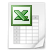### Lozenge method of John Horton Conway

With the Lozenge method of John Horton Conway you get magic squares of odd order and you find all odd numbers in the (white) 'diamond' and all even numbers outside the diamond (in the dark area). The Lozenge method looks like the diagonal method.

Put the odd numbers in rows coss & upwards in the magic square. Start with number 1 exactly in the middle of the left column.

 3 1 3 1 5 1 15 9 5 5 3 3 15 1 5 9 7 7

The remaining (even) numbers are 2, 4, 6 and 8. In the left column the magic sum needs +14 and 6+8=14. Put 8 in the bottom and you get the sum of 15 in the bottom row with only two numbers. So you must put 8 in top.

 15 15 9 13 11 11 8 3 15 1 5 9 13 6 7

In the top row you need 4 and in the bottom row you need 2, so you know where to put the numbers 4 and 2.

 15 15 15 15 15 15 8 3 4 15 1 5 9 15 6 7 2

Use the Lozenge method to construct magic squares of odd order (= 3x3, 5x5, 7x7, ... magic square).3x3, Lozenge method.xls